### 饼图

 `12345678910111213` `````` import pandas as pd import numpy as np import matplotlib.pyplot as plt def craw_pie(): series = pd.Series(3 * np.random.rand(4), index=["1", "2", "3", "4"], name="series") series.plot.pie(figsize=(6, 6)); plt.show() if __name__ == '__main__': craw_pie() ``````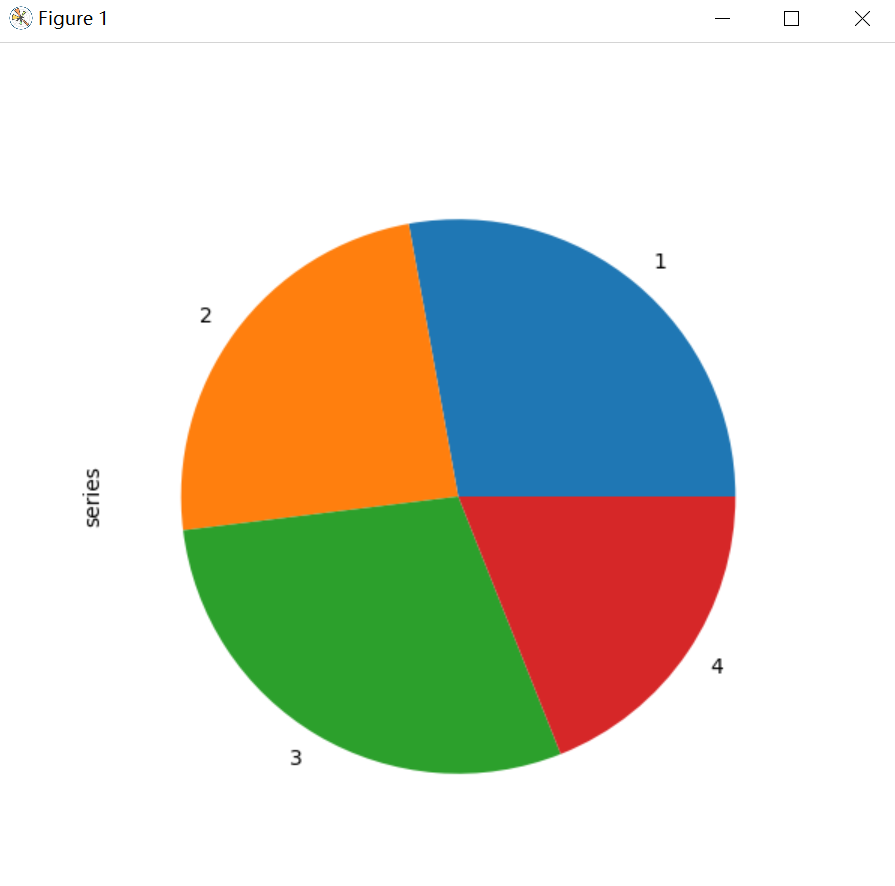*注意:* 带DataFrame的饼图要求您通过y参数指定目标列，或者subplot =True。 当指定y时，将绘制选定列的饼图。 如果指定subplot =True，则将每个列的饼图绘制为子图。 默认情况下，将在每个饼图中绘制一个图例; 指定legend=False来隐藏它。

 `12345678910111213` `````` import pandas as pd import numpy as np import matplotlib.pyplot as plt def draw_pie1(): df = pd.DataFrame( 3 * np.random.rand(4, 2), index=["a", "b", "c", "d"], columns=["x", "y"]) df.plot.pie(subplots=True, figsize=(8, 4)) plt.show() if __name__ == '__main__': draw_pie1() ``````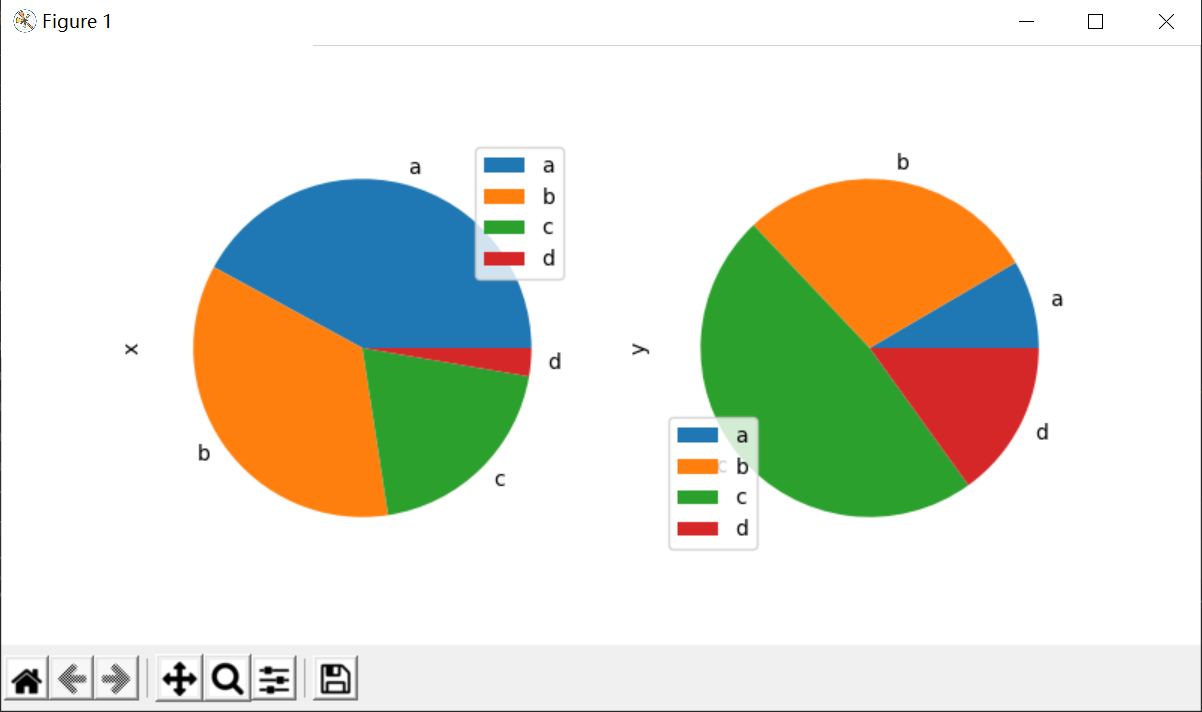`12345678910111213` `````` import pandas as pd import numpy as np import matplotlib.pyplot as plt def draw_pie1(): df = pd.DataFrame( 3 * np.random.rand(4, 2), index=["a", "b", "c", "d"], columns=["x", "y"]) df.plot.pie(subplots=True, figsize=(8, 4), legend=False) plt.show() if __name__ == '__main__': draw_pie1() ``````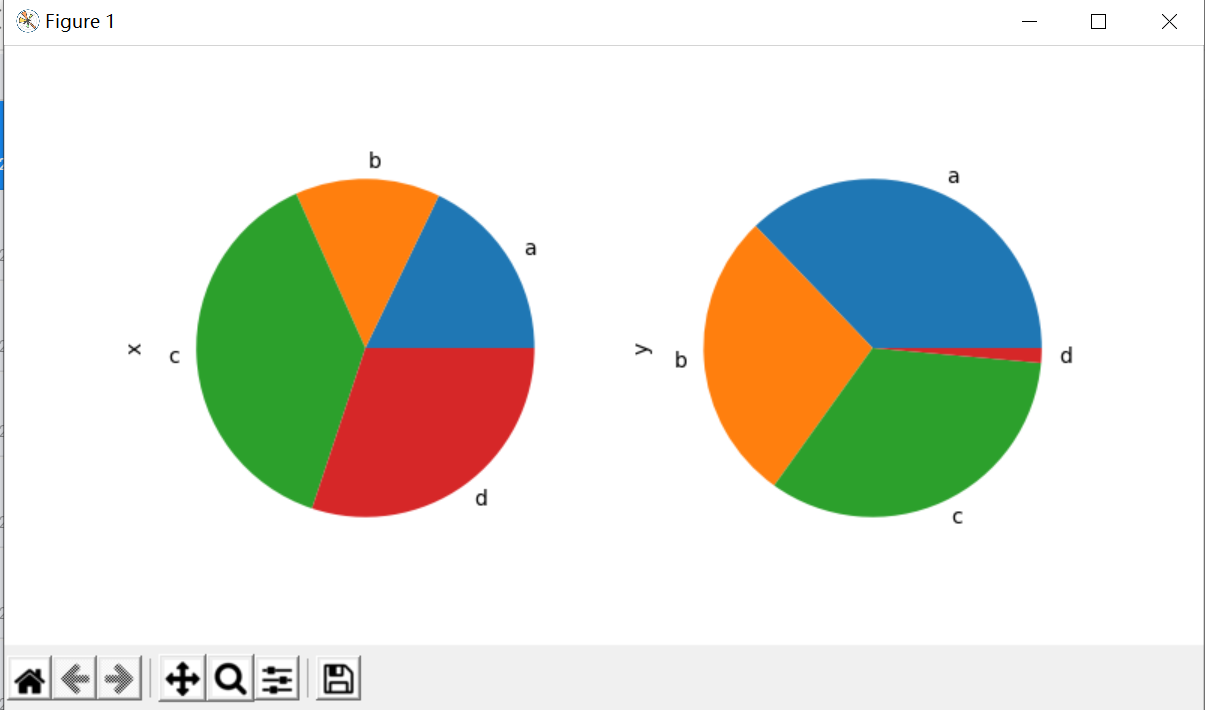`12345678910111213` `````` def draw_pie2(): series = pd.Series(3 * np.random.rand(4), index=["1", "2", "3", "4"], name="series") series.plot.pie( labels=["A", "B", "C", "D"], colors=["r", "g", "b", "c"], autopct="%.2f", fontsize=20, figsize=(6, 6),) plt.show() if __name__ == '__main__': draw_pie2() ``````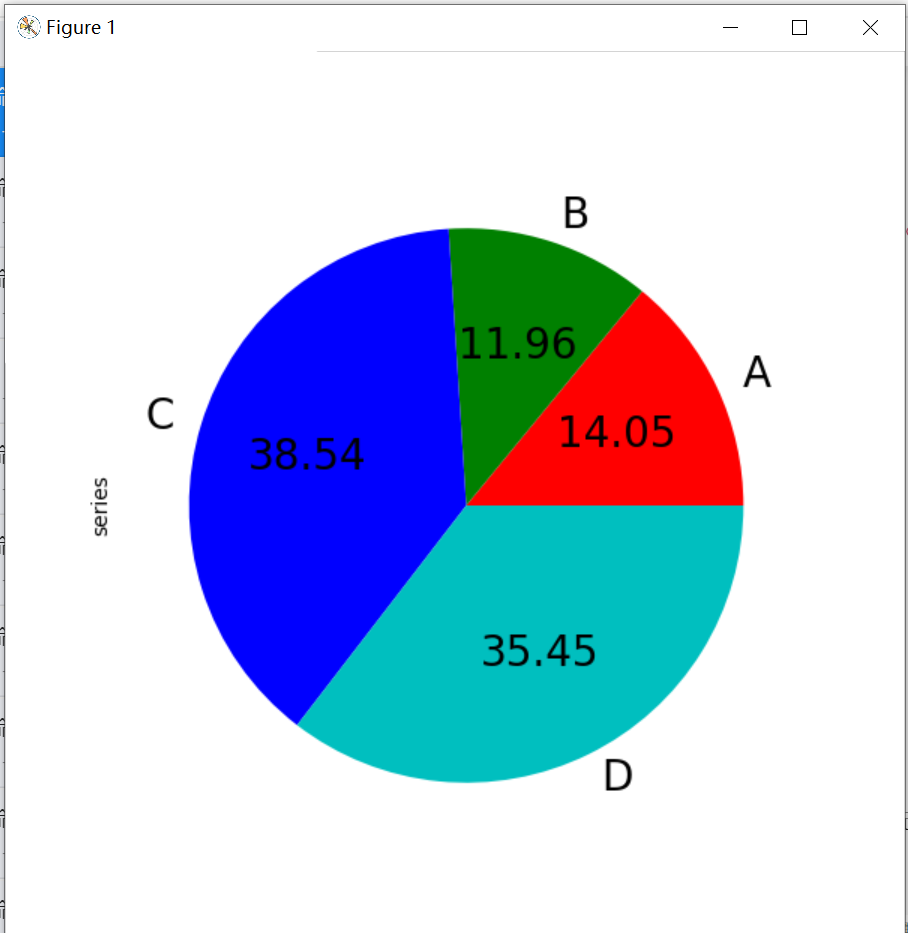`1234567` ``````def draw_pie3(): series = pd.Series([0.1] * 4, index=["a", "b", "c", "d"], name="series2") series.plot.pie(figsize=(6, 6)) plt.show() if __name__ == '__main__': draw_pie3() ``````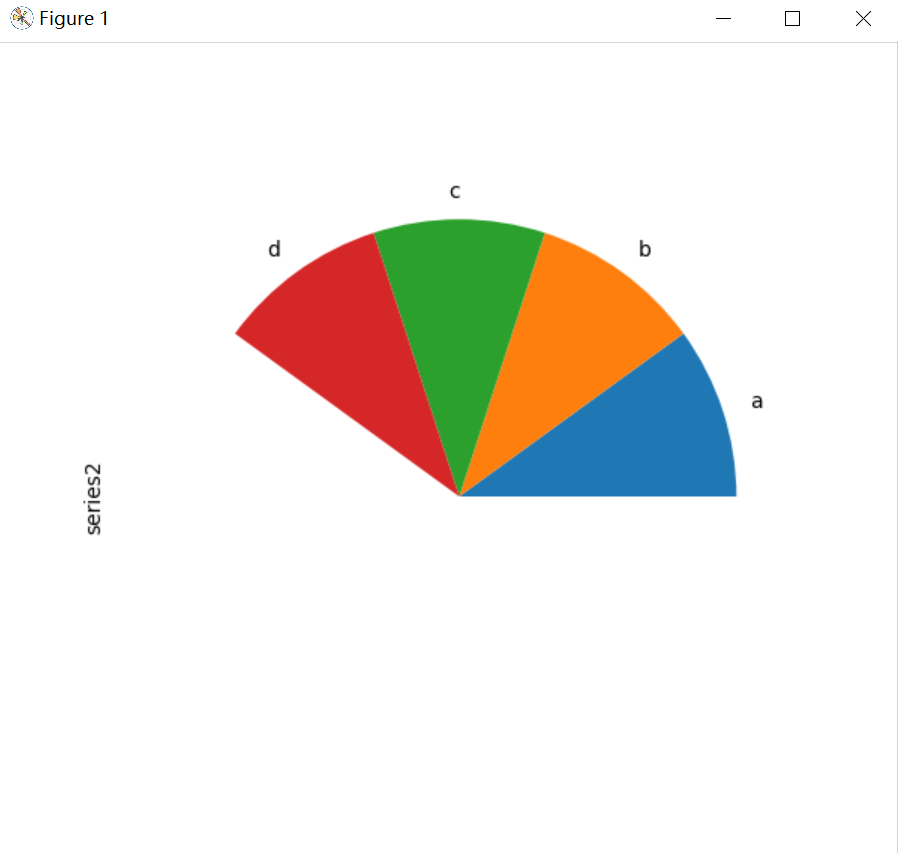### 散射矩阵图

 `12345678910111213` ``````import pandas as pd import numpy as np import matplotlib.pyplot as plt from pandas.plotting import scatter_matrix def draw_pie4(): df = pd.DataFrame(np.random.randn(1000, 4), columns=["a", "b", "c", "d"]) scatter_matrix(df, alpha=0.2, figsize=(6, 6), diagonal="kde") plt.show() if __name__ == '__main__': draw_pie4() ``````

### 总结